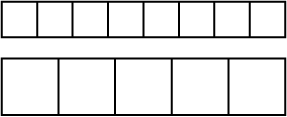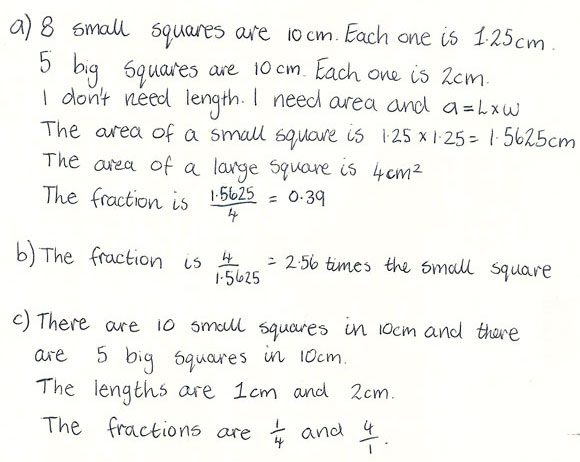# Rows of squares

A year 8 student response to a fractions task illustrates very competent reasoning about a complex question. The set task asks for reasoning, not just an answer. This allows the teacher to observe and question students about their reasoning processes before the responses are written in a more formal fashion.

The small squares in the first row are all the same size. Imagine that when put together like this, they measure 10 cm.

The larger squares in the second row are also all the same size. Together, they also measure 10 cm.a) What fraction of the area of one large square would a small square be? Explain your answer.

b) What fraction of the area of a small square would a large square be? Write your reasoning.

c) Make up a problem that is similar to this and explain how you would work it out.

### Sample student response